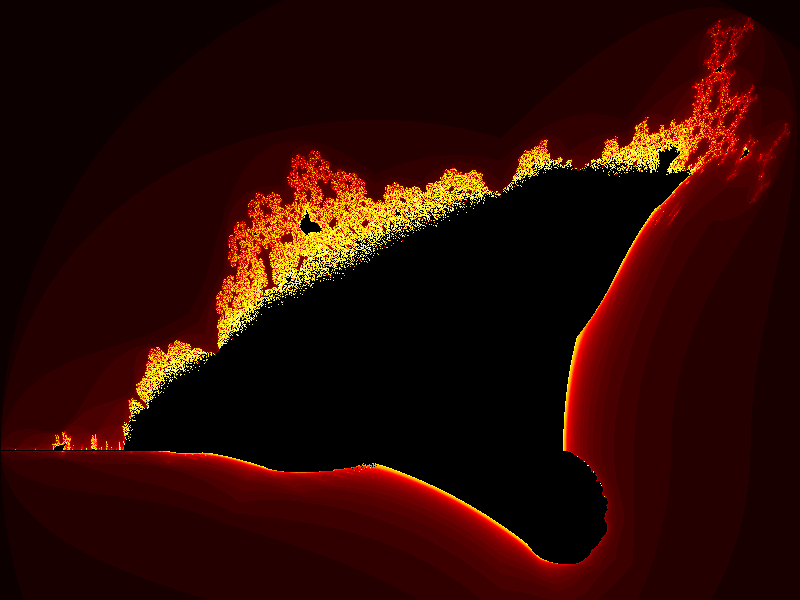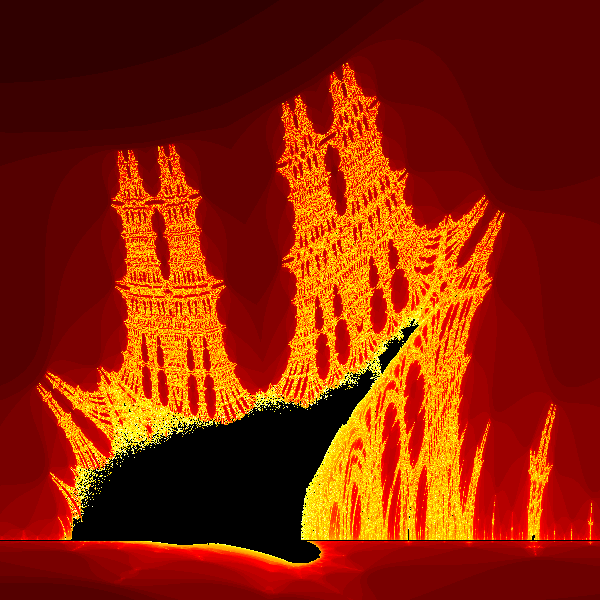# Burning ship fractal in generativepy

Martin McBride
2021-12-15

The burning ship fractal is another famous fractal that can be implemented easily with generativepy.

Like the Mandelbrot set, the burning ship is an escape-time fractal.

## Burning ship formula

The burning ship equations are similar to the Mandelbrot equations:

xnext = x*x - y*y + c1
ynext = 2*abs(x*y) + c2


Where c1 and c2 are two constant values.

The difference compared to the Mandelbrot equations is that the ynext equation uses abs(x*y) rather than just x*y. The absolute value is the positive size of the number, ignoring its sign. So abs(3) is 3, abs(-3) is also 3.

Here is the image created:## The full code

Here is the full code for the burning ship fractal:

from generativepy.bitmap import Scaler
from generativepy.nparray import (make_nparray_data, make_npcolormap, save_nparray,
from generativepy.color import Color
from generativepy.utils import temp_file
import numpy as np

MAX_COUNT = 256

def calc(c1, c2):
x = y = 0
for i in range(MAX_COUNT):
x, y = x*x - y*y + c1, abs(2*x*y) + c2
if x*x + y*y > 4:
return i + 1
return 0

def paint(image, pixel_width, pixel_height, frame_no, frame_count):
scaler = Scaler(pixel_width, pixel_height, width=3.2, startx=-2, starty=-1.8)

for px in range(pixel_width):
for py in range(pixel_height):
x, y = scaler.device_to_user(px, py)
count = calc(x, y)
image[py, px] = count

def colorise(counts):
counts = np.reshape(counts, (counts.shape, counts.shape))

colormap = make_npcolormap(MAX_COUNT+1,
[Color('black'), Color('red'), Color('orange'), Color('yellow'), Color('white')],
[16, 8, 32, 128])

outarray = np.zeros((counts.shape, counts.shape, 3), dtype=np.uint8)
apply_npcolormap(outarray, counts, colormap)
return outarray

data = make_nparray_data(paint, 800, 600, channels=1)

filename = temp_file('burning-ship.dat')
save_nparray(filename, data)

frame = colorise(data)

save_nparray_image('burning-ship.png', frame)


This code is available on github in blog/fractals/burning_ship.py.

As well as changing the formula we have also slightly adjusted the area of the user space (in the call to Scaler), to better fit the image. In addition, we have used a red, orange, and yellow colour scheme.

## Zooming in

Just like the Mandelbrot set, the burning ship fractal contains miniature "copies" of itself at various places. They aren't actual copies, they have different but similar shapes.

The small black blob to the left of the main feature is arguably more impressive than the main shape and is the real reason for the name. Here is what it looks like zoomed in:To obtain this image, we can use the code above by simple altering the scaler parameters in the paint function:

    scaler = Scaler(pixel_width, pixel_height, width=0.1, startx=-1.8, starty=-0.09)


These parameters zoom in on the new area.